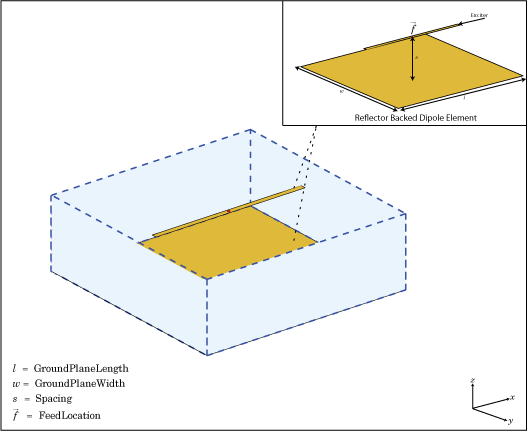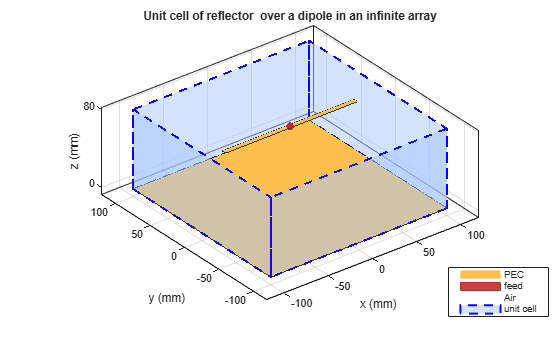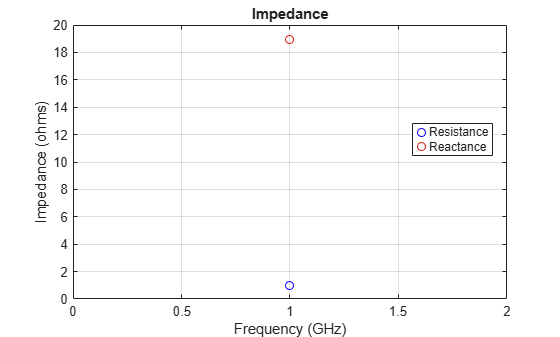# infiniteArray

Create 2-D custom mesh antenna on X-Y plane

## Description

The `infiniteArray` object is an infinite antenna array in the X-Y plane. Infinite array models a single antenna element called the unit cell. Ground plane of the antennas specifies the boundaries of the unit cell. Antennas without a ground plane require a reflector. By default, the infinite array has reflector-backed dipoles as antenna elements. The default dimensions are chosen for an operating frequency of 1 GHz.## Creation

### Description

``` `infa = infiniteArray` creates an infinite antenna array in the X-Y plane. ```

example

``` `infa = infiniteArray(Name,Value)` creates an infinite antenna array with additional properties specified by one, or more name-value pair arguments. `Name` is the property name and `Value` is the corresponding value. You can specify several name-value pair arguments in any order as `Name1, Value1, ..., NameN, ValueN`. Properties not specified retain default values.```

## Properties

expand all

Type of individual antenna elements in unit cell, specified as an object. Antenna without a groundplane is backed using a reflector. The ground plane size specifies the unit cell boundaries.

Example: `'Element',reflector`

Scan direction in azimuth plane, specified as a scalar in degrees.

Example: `'ScanAzimuth',25`

Data Types: `double`

Scan direction in elevation plane, specified as a scalar in degrees.

Example: `'ScanElevation',80`

Data Types: `double`

Remove the ground plane of the reflector with air substrate, specified as a numeric or logical `1` (`true`) or `0` (`false`). When you specify `1`, the object removes the ground plane. When you specify `0`, the object does not remove the ground plane

Example: `'RemoveGround',1`

Data Types: `logical`

## Object Functions

 `numSummationTerms` Change number of summation terms for calculating periodic Green's function `show` Display antenna or array structure; display shape as filled patch `info` Display information about antenna or array `axialRatio` Axial ratio of antenna `beamwidth` Beamwidth of antenna `charge` Charge distribution on metal or dielectric antenna or array surface `correlation` Correlation coefficient between two antennas in array `current` Current distribution on metal or dielectric antenna or array surface `design` Design prototype antenna or arrays for resonance around specified frequency `EHfields` Electric and magnetic fields of antennas; Embedded electric and magnetic fields of antenna element in arrays `impedance` Input impedance of antenna; scan impedance of array `layout` Display array or PCB stack layout `mesh` Mesh properties of metal or dielectric antenna or array structure `pattern` Radiation pattern and phase of antenna or array; Embedded pattern of antenna element in array `patternAzimuth` Azimuth pattern of antenna or array `patternElevation` Elevation pattern of antenna or array `rcs` Calculate and plot radar cross section (RCS) of platform, antenna, or array `returnLoss` Return loss of antenna; scan return loss of array `sparameters` Calculate S-parameter for antenna and antenna array objects

## Examples

collapse all

Create an infinite array with reflector-backed dipoles as unit cells. Scan the array at boresight. Visualize the unit cell.

```infa = infiniteArray('Element',reflector,'ScanAzimuth',0, ... 'ScanElevation',90); show(infa)```Calculate the scan impedance of an infinite array at 1GHz. To calculate the impedance, scan the infinite array from boresight to horizon in the elevation plane.

```infa = infiniteArray; theta0deg = linspace(0,90,5); zscan = nan(1,numel(theta0deg)); for j = 1:numel(theta0deg) infa.ScanElevation = theta0deg(j); zscan(1,j) = impedance(infa,1e9); end plot(zscan)```Calculate the scan impedance at bore sight of a metal infinite array without ground plane at a frequency of 1 GHz.

```h = infiniteArray('Element', reflector, 'ScanAzimuth', 0, ... 'ScanElevation', 90, 'RemoveGround', 1); zin = impedance(h,1e9)```
```zin = 48.2919 + 13.1800i ```
`impedance(h,1e9)`Plot the scan impedance at the boresight of a metal-dielectric infinite array at a frequency of 1 GHz.

```ant = patchMicrostrip('Substrate',dielectric('Teflon')); h = infiniteArray('Element',ant,'ScanAzimuth',0,'ScanElevation',90); impedance(h,1e9)```Balanis, C.A. Antenna Theory: Analysis and Design. 3rd Ed. New York: Wiley, 2005.